Next: Two-Dimensional Uniform Flow Up: Two-Dimensional Incompressible Inviscid Flow Previous: Two-Dimensional Flow

# Velocity Potentials and Stream Functions

As we have seen, a two-dimensional velocity field in which the flow is everywhere parallel to the-plane, and there is no variation along the-direction, takes the form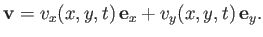(5.16)

Moreover, if the flow is irrotational then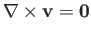is automatically satisfied by writing, whereis termed the velocity potential. (See Section 4.15.) Hence,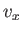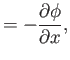(5.17)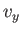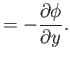(5.18)

On the other hand, if the flow is incompressible then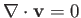is automatically satisfied by writing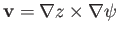, where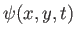is termed the stream function. (See Section 5.2.) Hence,(5.19)(5.20)

Finally, if the flow is both irrotational and incompressible then Equations (5.17)-(5.18) and (5.19)-(5.20) hold simultaneously, which implies that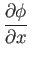(5.21)(5.22)

It immediately follows, from the previous two expressions, that(5.23)

or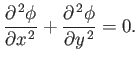(5.24)

Likewise, it can also be shown that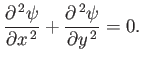(5.25)

We conclude that, for two-dimensional, irrotational, incompressible flow, the velocity potential and the stream function both satisfy Laplace's equation. Equations (5.21) and (5.22) also imply that(5.26)

In other words, the contours of the velocity potential and the stream function cross at right-angles.Next: Two-Dimensional Uniform Flow Up: Two-Dimensional Incompressible Inviscid Flow Previous: Two-Dimensional Flow
Richard Fitzpatrick 2016-03-31